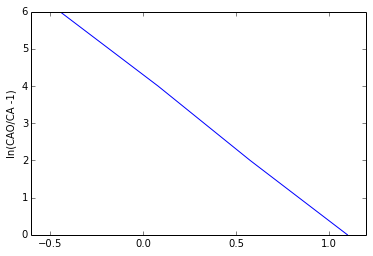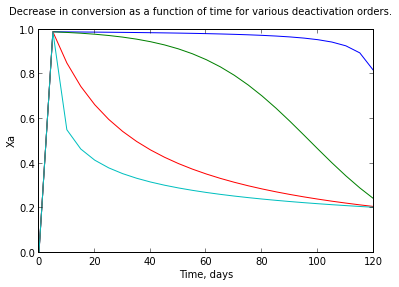Chapter 21 : The Rate and Performance Equations¶

Example 21.1 page no : 486¶

In :
%matplotlib inline

import math
from numpy import *
from matplotlib.pyplot import *
from scipy import stats

# Variables
t = [0,2,4,6];                 # time
XA = [0.75,0.64,0.52,0.39];    # XA
t1 = 4000.                      #kg.s/m3
density_s = 1500.               #kg/m3
De = 5.*10**-10;
d = 2.4*10**-3;

# Calculations
y = zeros(4)
for i in range(4):
y[i] = math.log((1./(1-XA[i]))-1);

plot(y,t)
ylabel("ln(CAO/CA -1)")

coeff  = stats.linregress(t,y);
kd = coeff;
k = math.exp(coeff)/t1;
L = d/6;
Mt = L*math.sqrt(k*density_s/De);
#Assuming Runs were made in regime of strong resismath.tance to  pore diffusion

k1 = ((math.exp(coeff))**2)*(L**2)*density_s/(t1*t1*De);
kd1 = -2*coeff;
Mt = L*math.sqrt(k1*density_s/De);

# Results
print " Rate equation in diffusion free regime with deactivation is %.2f  m**3/kg.s"%(k1)
print " CA*a with  -da/dthr-1  is %.2f a,hr**-1"%(kd1)

#In strong pore diffusion
k2 = k1*math.sqrt(De/(k1*density_s));
print " CA*a**0.5/L with  -da/dthr-1 is %.2f a,hr**-1"%(kd1),
Populating the interactive namespace from numpy and matplotlib
Rate equation in diffusion free regime with deactivation is 0.27  m**3/kg.s
CA*a with  -da/dthr-1  is 0.51 a,hr**-1
CA*a**0.5/L with  -da/dthr-1 is 0.51 a,hr**-1
WARNING: pylab import has clobbered these variables: ['draw_if_interactive']
%pylab --no-import-all prevents importing * from pylab and numpyExample 21.2 pageno : 492¶

In :
import math
from matplotlib.pyplot import *
from numpy import *

# Variables
PAo = 3.                #atm
R = 82.06*10**-6        #m3.atm/mol.k
T = 730.                #k
W = 1000.               #kg
FAo = 5000.             #mol/hr

# Calculations
CAo = PAo/(R*T);
tau = W*CAo/FAo;
i = 0;

a = zeros(25)
XA = zeros(25)
a1 = zeros(25)
XA1 = zeros(25)
a2 = zeros(25)
XA2 = zeros(25)
a3 = zeros(25)
XA3 = zeros(25)
a = linspace(0,120,25)
for t in xrange(0,120,5):
i = i+1;
#Part a
a[i] = 1-(8.3125*10**-3)*t;
XA[i] = (tau**2)*a[i]/(1+(tau**2)*a[i]);
#Part b
a1[i] = math.exp(-0.05*t);
XA1[i] = (tau**2)*a1[i]/(1+(tau**2)*a1[i]);
#Part c
a2[i] = 1/(1+3.325*t);
XA2[i] = (tau**2)*a2[i]/(1+(tau**2)*a2[i]);
#Part d
a3[i] = 1/(math.sqrt(1+1333*t));
XA3[i] = (tau**2)*a3[i]/(1+(tau**2)*a3[i]);

t = linspace(0,120,25)
plot(t,XA,t,XA1,t,XA2,t,XA3)
suptitle("Decrease in conversion as a function of time for various deactivation orders.")
xlabel("Time, days")
ylabel("Xa")

def f13(t):
return ((100*(1-(8.3125*10**-3)*t))/(1+100*(1-(8.3125*10**-3)*t)))

def f14(t):
return (100.*math.exp(-0.05*t))/(1+100*math.exp(-0.05*t))

def f15(t):
return ((100*(1./(1+3.325*t)))/(1+100*(1/(1+3.325*t))))

def f16(t):
return ((100*1./(math.sqrt(1+1333*t)))/(1+100*(1/math.sqrt(1+1333*t))))

# Results
print " for d = 0,the mean conversion is % f"%(XA_avg)
print " for d = 1,the mean conversion is % f"%(XA1_avg)
print " for d = 2,the mean conversion is % f"%(XA2_avg)
print " for d = 3,the mean conversion is % f"%(XA3_avg)

show()
for d = 0,the mean conversion is  0.955970
for d = 1,the mean conversion is  0.732280
for d = 2,the mean conversion is  0.400874
for d = 3,the mean conversion is  0.298840In [ ]: